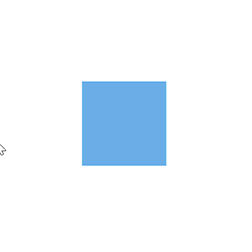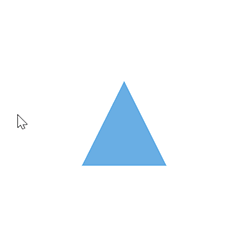Search results

# Interaction in Blazor Diagram component

03 Aug 2021 / 5 minutes to read

Diagram provides the support to drag, resize, or rotate the node interactively.

## Select

A node can be select at runtime by using the `Select` method and clear the selection in the diagram using the `ClearSelection`. The following code explains how to select and clear selection in the diagram.

``````@using Syncfusion.Blazor.Diagrams
@using System.Collections.ObjectModel

<input type="button" value="Select" @onclick="OnSelect">
<input type="button" value="UnSelect" @onclick="@UnSelect" />
<SfDiagram @ref="@Diagram" Height="600px" Nodes="@NodeCollection">
</SfDiagram>

@code {
// reference of the diagram
SfDiagram Diagram;

// To define node collection
public ObservableCollection<DiagramNode> NodeCollection = new ObservableCollection<DiagramNode>() { };
protected override void OnInitialized()
{
// A node is created and stored in nodes collection.
DiagramNode node1 = new DiagramNode()
{
// Position of the node
OffsetX = 250, OffsetY = 250,
// Size of the node
Width = 100, Height = 100,
Style = new NodeShapeStyle() { Fill = "#6BA5D7", StrokeColor = "white" }
};
}
public void OnSelect()
{
// Select the node
Diagram.Select(new ObservableCollection<DiagramNode>() { Diagram.Nodes }, null);
}
public void UnSelect()
{
// clear selection in the diagram
Diagram.ClearSelection();
}
}``````

And also the selection enable during the interaction.

• An element can be selected by clicking that element.
• When you select the elements in the diagram, the `SelectionChanged` event gets triggered and to do customization in this event.## Drag

A node can be drag at runtime by using the `Drag` method. The following code explains how to drag the node by using the drag method.

``````@using Syncfusion.Blazor.Diagrams
@using System.Collections.ObjectModel

<input type="button" value="Drag" @onclick="OnDrag">
<SfDiagram @ref="@Diagram" Height="600px" Nodes="@NodeCollection">
</SfDiagram>

@code {
// reference of the diagram
SfDiagram Diagram;
// To define node collection
public ObservableCollection<DiagramNode> NodeCollection = new ObservableCollection<DiagramNode>() { };
protected override void OnInitialized()
{
// A node is created and stored in nodes collection.
DiagramNode node1 = new DiagramNode()
{
// Position of the node
OffsetX = 250, OffsetY = 250,
// Size of the node
Width = 100, Height = 100,
Style = new NodeShapeStyle() { Fill = "#6BA5D7", StrokeColor = "white" }
};
}
public void OnDrag()
{
// Drag the node
Diagram.Drag(Diagram.Nodes, 10, 10);
}
}``````

And also the drag the node during the interaction.

• An object can be dragged by clicking and dragging it. When multiple elements are selected, dragging any one of the selected elements move all the selected elements.
• When you drag the elements in the diagram, the `OnPositionChange` event gets triggered and to do customization in this event.## Resize

A node can be resize at runtime by using the `Scale` method. The following code explains how to resize the node by using the scale method.

``````@using Syncfusion.Blazor.Diagrams
@using System.Collections.ObjectModel

<input type="button" value="Resize" @onclick="OnResize">
<SfDiagram @ref="@Diagram" Height="600px" Nodes="@NodeCollection">
</SfDiagram>

@code {
// reference of the diagram
SfDiagram Diagram;
// To define node collection
public ObservableCollection<DiagramNode> NodeCollection = new ObservableCollection<DiagramNode>() { };
protected override void OnInitialized()
{
// A node is created and stored in nodes collection.
DiagramNode node1 = new DiagramNode()
{
// Position of the node
OffsetX = 250, OffsetY = 250,
// Size of the node
Width = 100, Height = 100,
Style = new NodeShapeStyle() { Fill = "#6BA5D7", StrokeColor = "white" }
};
}
public void OnResize()
{
// Resize the node
Diagram.Scale(Diagram.Nodes, 0.5, 0.5, new PointModel() { X = 0, Y = 0 } );
}
}``````

And also the resize the node during the interaction.

• Selector is surrounded by eight thumbs. When dragging these thumbs, the selected items can be resized.
• When one corner of the selector is dragged, the opposite corner will be in a static position.
• When a node is resized, the `OnSizeChange` event gets triggered.Note: While dragging and resizing, the objects are snapped towards the nearest objects to make better alignments. For better alignments, refer to `Snapping`.

## Rotate

A node can be rotate at runtime by using the `Rotate` method. The following code explains how to rotate the node by using method.

``````@using Syncfusion.Blazor.Diagrams
@using System.Collections.ObjectModel

<input type="button" value="Rotate" @onclick="OnRotate">
<SfDiagram @ref="@Diagram" Height="600px" Nodes="@NodeCollection">
</SfDiagram>

@code {
// reference of the diagram
SfDiagram Diagram;

// To define node collection
public ObservableCollection<DiagramNode> NodeCollection = new ObservableCollection<DiagramNode>() { };
protected override void OnInitialized()
{
// A node is created and stored in nodes collection.
DiagramNode node1 = new DiagramNode()
{
// Position of the node
OffsetX = 250, OffsetY = 250,
// Size of the node
Width = 100, Height = 100,
Style = new NodeShapeStyle() { Fill = "#6BA5D7", StrokeColor = "white" }
};
}
public void OnRotate()
{
// Rotate the node
Diagram.Rotate(Diagram.Nodes, Diagram.Nodes.RotateAngle+10);
}
}``````

And also the rotate the node during the interaction.

• A rotate handler is placed above the selector. Clicking and dragging the handler in a circular direction lead to rotate the node.
• The node is rotated with reference to the static pivot point.
• Pivot thumb (thumb at the middle of the node) appears when rotating the node to represent the static point.For more information about node interaction, refer to `Node Interaction`.